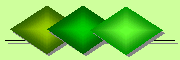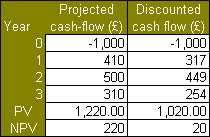De Claron LtdExpress Accountancy services at affordable prices
 Accounting Property Investment Reporting Company Secretarial ServicesHelp

Present Discounted Value

Present discounted value, is the value on a given date of a future payment or series of future payments, discounted to reflect the time value of money and other factors such as investment risk.

Time value of money
The time value of money is value of money with any additional interest it may generate over time. For example, \$100 which is invested at 10% interest per annum is worth \$110 in one year time. However, in today's terms it is currently worth only \$100.

Investment risk
Investment risk is the risk of uncertainty in realising a return on an investment or even incurring potential financial loss on it. For example, instead of earning the expected 10% per annum interest on \$100, one earns 2% per annum giving only \$102 in a year time instead of the anticipated \$110. Or, alternatively, one receives only a total of \$90 in a year time in which case the investment of \$100 has actually generated a loss of \$10.

The above can be illustrated by a simple investment project which has a capital outlay of \$1,000 and is expected to generate \$1,220 over the next 3 years. In the first year the expected return is \$410. However, the present value (PV) has been discounted to \$317 to reflect the time value and investment risk of the project. This is pattern is repeated for the remaining 2 years where the present value is always less than the expected value for each subsequent year for the project. Given this, the present value of the return from the project at the end of 3 years is \$1,020 which is \$200 less than the exepected value of 1,220. This leaves a net present value (NPV) of \$20 which is the amount discounted from the \$220 expected at the end of the project.